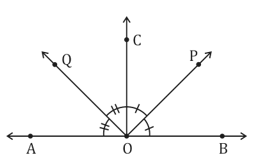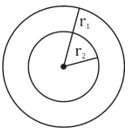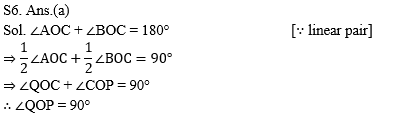Dear Students!!! There is most general as well as a scoring section in all the competitive entrance examinations in the teaching field i.e "Mathematics".Because in this section only one thing is work i.e your accuracy and that could be nourished with the daily practice. So, for this, we are providing you the daily quiz for all teaching exams i.e CTET Exam 2019DSSSB ,KVS,STET Exam.

Q1. Tonu and Vishu start walking together on a circular path in opposite directions. If they meet after some time at a particular point, what is the sum of distances covered by them? Give that r is the radius of path.

(a) 2πr
(b) πr
(c) 4πr
(d) None of these

Q2. Which of the following groups of angles represents the angles of a triangle?

(a) 45°, 55°, 60°
(b) 50°, 60°, 70°
(c) 50°, 70°, 55°
(d) 65°, 70°, 75°

Q3. A triangular prism is usually as the shape of a kaleidoscope. It’s base and top are triangles. How many faces are there in it?

(a) Four
(b) Five
(c) Six
(d) Seven

Q4. In a pair of concentric circles, if radii of outer and inner circles are r₁ and r₂ respectively, which of the following is true?

(a) r₁ + r₂ = 0
(b) r₁ > r₂
(c) r₁ < r₂
(d) r₁ = r₂

Q5. OA and OB are opposite rays and a ray OC is inclined. If one of the angles is 75°, the measurement of the other angle is

(a) 105°
(b) 70°
(c) 15°
(d) None of these

Q6. In figure, OP bisects ∠BOC and OQ bisects ∠AOC, then ∠POQ is(a) 90°
(b) 75°
(c) 105°
(d) 80°

Q7. Which of the following statements is false?

(a) A line segment can be produced to any desired length
(b) Through a given point, only one straight line can be drawn
(c) Through two given points, it is possible to draw one and only one straight line
(d) Two straight lines can intersect in only one point

Q8. In the given figure, if ∠COE = 90°, then the value of x is(a) 120°
(b) 60°
(c) 45°
(d) 30°

Q9. In the given figure, AB and CD are two parallel lines. A line XY meets the lines AB and CD at E and F, respectively. If ∠XEA = 110°, then ∠EFD is equal to(a) 110°
(b) 70°
(c) 80°
(d) 45°

Q10. ABC is a triangle and DE is drawn parallel to BC cutting the other sides at D and E. If AB = 3.6 cm, AC = 2.4 cm, AD = 2.1 cm, then AE is equal to

(a) 1.4 cm
(b) 1.2 cm
(c) 1.8 cm
(d) 1.05 cm

Solutions

S1. Ans.(a)
Sol. Let Tonu and Vishu start walking from the point A and meet each other at the point B. Then, clearly the sum of distances covered by both of them is equal to the circumference of the path which is given by 2πr.

S2. Ans.(b)
Sol. Sum of the angles of option (b) is equal to 180°
i.e., 50° + 60° + 70° = 180°
Thus, this group represents the angles of a triangle.

S3. Ans.(b)
Sol. A triangular prism has five faces.

S4. Ans.(b)
Sol. If r₁ and r₂ be the radii of outer and inner circles respectively, then r₁ > r₂.S5. Ans.(a)
Sol. Since, OA and OB are opposite rays.
⇒ AB is a line.
Sum of the two angles = 180°
∴ Second angle = 180° – 75° = 105°S7. Ans.(b)
Sol. An infinite number of straight lines can be drawn through a given point.

S8. Ans.(d)
Sol. Since, AOB is a line
∴ ∠AOB = 180°
⇒ ∠AOC + ∠COE + ∠EOB = 180°
⇒ 2x + 90° + x = 180°
⇒ 3x = 90° ⇒ x = 30°

S9. Ans.(b)
Sol ∵ ∠XEA = ∠BEF = 110° [vertically opposite angles]
∠BEF + ∠EFD = 180° [consecutive interior angles]
∴ ∠EFD = 180° – 110° = 70°You may also like to read :